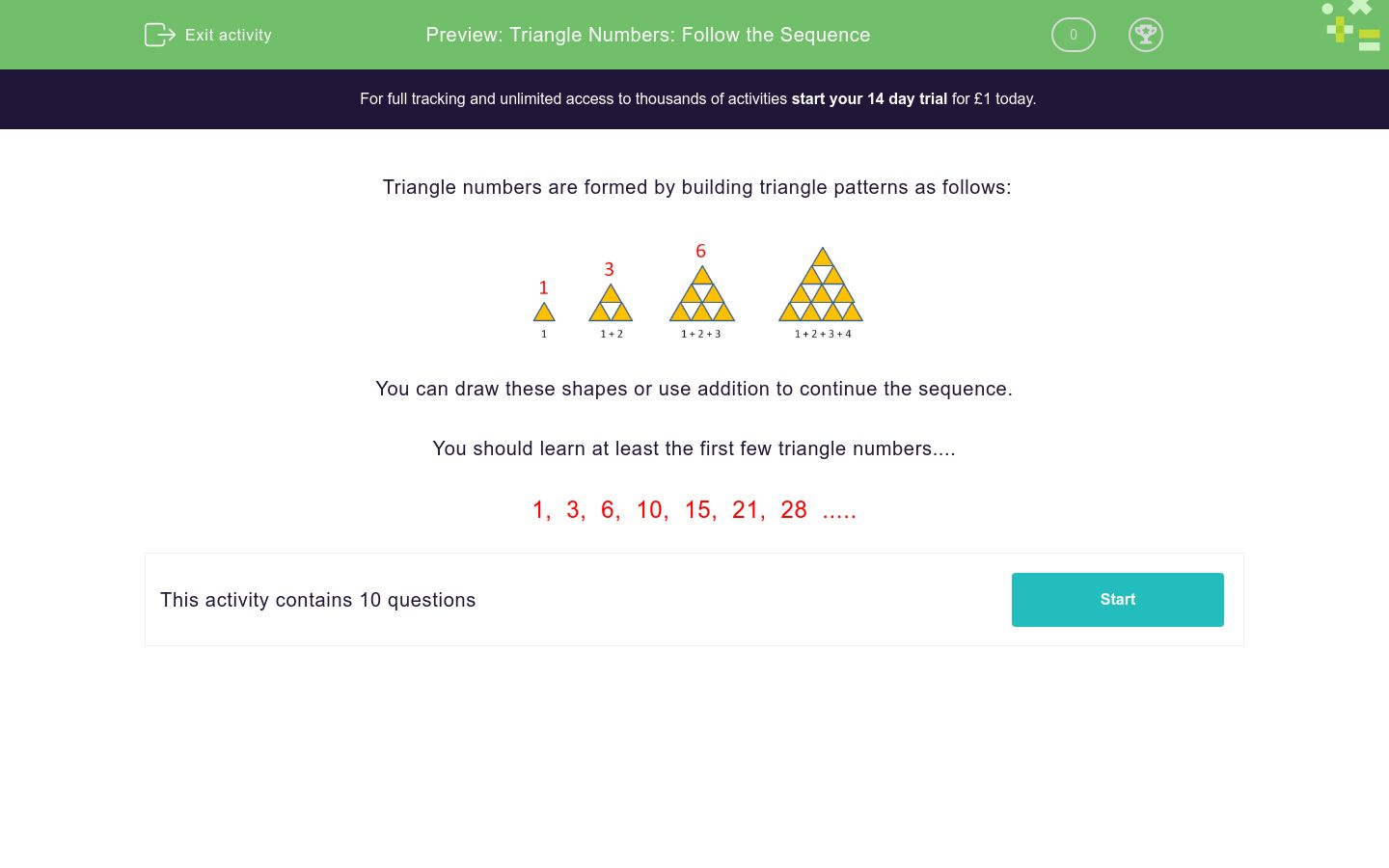# Triangle Numbers: Follow the Sequence

In this worksheet, students recognise the sequence of triangles and add in missing numbers.Key stage:  KS 2

Curriculum topic:   Number: Number and Place Value

Curriculum subtopic:   Solve Number Problems to 1 000 000

Difficulty level:### QUESTION 1 of 10

Triangle numbers are formed by building triangle patterns as follows:You can draw these shapes or use addition to continue the sequence.

You should learn at least the first few triangle numbers....

1,  3,  6,  10,  15,  21,  28  .....

Is the following number a triangle number?

15

No

Yes

Is the following number a triangle number?

25

No

Yes

Is the following number a triangle number?

1

No

Yes

Is the following number a triangle number?

45

Yes

No

Is the following number a triangle number?

12

No

Yes

Is the following number a triangle number?

5

No

Yes

Is the following number a triangle number?

21

No

Yes

Is the following number a triangle number?

49

No

Yes

Is the following number a triangle number?

36

No

Yes

Is the following number a triangle number?

28

No

Yes

• Question 1

Is the following number a triangle number?

15

Yes
EDDIE SAYS
Following the pattern of triangle numbers, 15 is the fifth number in the sequence: 1 + 2 + 3 + 4 + 5 = 15
• Question 2

Is the following number a triangle number?

25

No
EDDIE SAYS
25 is not in the triangle number sequence.
• Question 3

Is the following number a triangle number?

1

Yes
EDDIE SAYS
Following the pattern of triangle numbers, 1 is the first number in the sequence.
• Question 4

Is the following number a triangle number?

45

Yes
EDDIE SAYS
Following the pattern of triangle numbers, 45 is the ninth number in the sequence: 1 + 2 + 3 + 4 + 5 + 6 + 7 + 8 + 9 = 45
• Question 5

Is the following number a triangle number?

12

No
EDDIE SAYS
12 is not in the triangle number sequence.
• Question 6

Is the following number a triangle number?

5

No
EDDIE SAYS
5 is not in the triangle number sequence.
• Question 7

Is the following number a triangle number?

21

Yes
EDDIE SAYS
Following the pattern of triangle numbers, 21 is the sixth number in the sequence: 1 + 2 + 3 + 4 + 5 + 6 = 21
• Question 8

Is the following number a triangle number?

49

No
EDDIE SAYS
49 is not in the triangle number sequence.
• Question 9

Is the following number a triangle number?

36

Yes
EDDIE SAYS
Following the pattern of triangle numbers, 36 is the eighth number in the sequence: 1 + 2 + 3 + 4 + 5 + 6 + 7 + 8 = 36
• Question 10

Is the following number a triangle number?

28

Yes
EDDIE SAYS
Following the pattern of triangle numbers, 28 is the seventh number in the sequence: 1 + 2 + 3 + 4 + 5 + 6 + 7 = 28
---- OR ----

Sign up for a £1 trial so you can track and measure your child's progress on this activity.

### What is EdPlace?

We're your National Curriculum aligned online education content provider helping each child succeed in English, maths and science from year 1 to GCSE. With an EdPlace account you’ll be able to track and measure progress, helping each child achieve their best. We build confidence and attainment by personalising each child’s learning at a level that suits them.

Get started